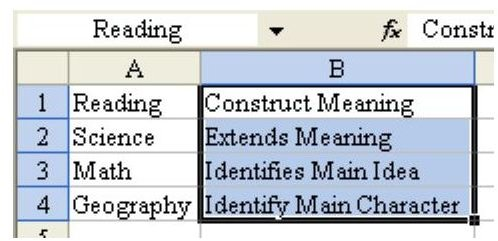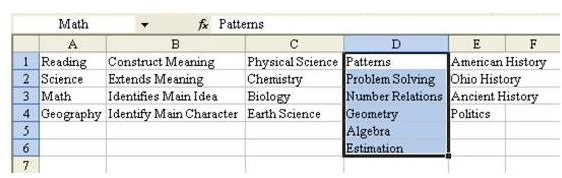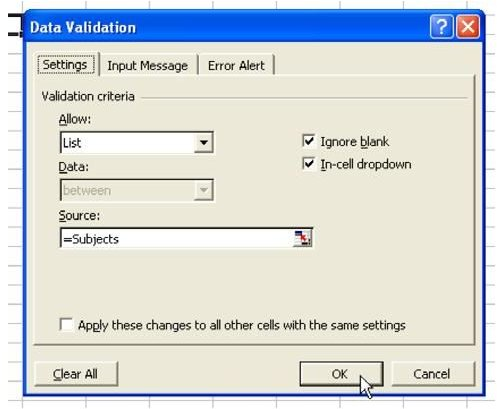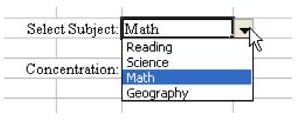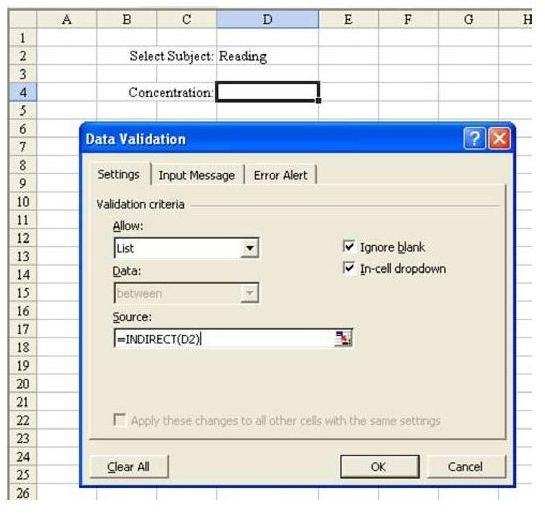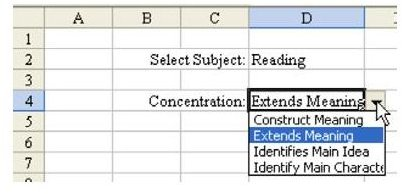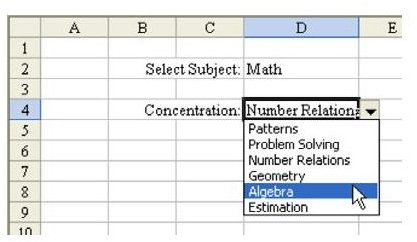# Learn How to Use Validation to Create Dependent Lists in Excel

Page content

Strategy: Use the INDIRECT function as the source of the second list.

1. On a blank sheet, set up a list of items for the first dropdown: Reading, Science, Math, and Geography. Name the range “Subjects”, as shown in Fig. 1501.

2. In another column, set up a list of choices available for reading.

3. Name this list Reading, as shown in Fig. 1502.

4. Repeat Step 3 for each item in list 1, as shown in Fig. 1503. In each case, the name of the new range must match the value in column A.

1. Set up the first dropdown list, where users will pick the subject. Select the cell. From the menu, select Data – Validation. Change the Allow box to be List; in the Source box, type =Subjects, as shown in Fig. 1504.

2. When you choose OK, cell D2 will have a dropdown list of subjects, as shown in Fig. 1505.

3. To set up the second dropdown, select cell D4. From the menu, select Data – Validation. Change the Allow: dropdown under Validation Criteria from Any Value to List. In the Source box, enter this formula: =INDIRECT(D2), as shown in Fig. 1506.

Result: When you select a value in D2, the formula for the second dropdown list will automatically update, as shown in Fig. 1507. The INDIRECT function looks in D2 and hopes to find a formula there. When they select Reading in D2, then the validation formula becomes =Reading. Since you cleverly set up a named range called Reading, Excel is able to populate the list.

When you change D2 to be Math, the =INDIRECT(D2) will become =Math. Again, since you have a named range called Math, Excel is able to fill in the second dropdown with Math subjects, as shown in Fig. 1508.

Summary: Using the INDIRECT function as the formula in the List for Data Validation will allow you to set up a second validation list that is dependent on the choice in the earlier list.

Commands Discussed: Data – Validation

Functions Discussed: =INDIRECT()

See all Microsoft Excel tips

## Images